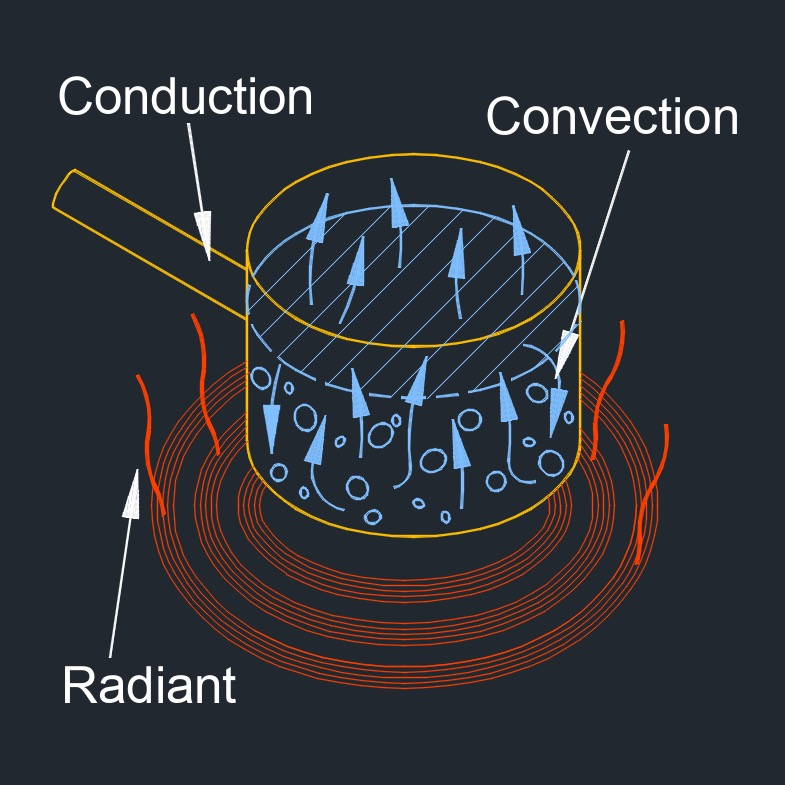# Condensate Load from Heating Liquid Batch

on . Posted in Fluid Dynamics## Condensate Load from Heating Liquid Batch formula

$$\large{ m_c = 1000 \; c_l \; SG_l \; V \; \frac{ T_o \;-\; T_i }{ L_s \; t_h } }$$
Symbol English Metric
$$\large{ m_c }$$ = condensate load  $$\large{lbm}$$  $$\large{kg}$$
$$\large{ t_h }$$ = heating time period $$\large{min}$$ $$\large{min}$$
$$\large{ L_s }$$ = latent heat of steam $$\large{\frac{Btu}{lbm}}$$ $$\large{\frac{kJ}{kg}}$$
$$\large{ SG_l }$$ = specific gravity of liquid $$\large{dimensionless}$$
$$\large{ c_l }$$ = specific heat of liquid $$\large{\frac{Btu}{lbm-F}}$$ $$\large{\frac{kJ}{kg-K}}$$
$$\large{ T_i }$$ = inlet temperature of liquid $$\large{F}$$ $$\large{K}$$
$$\large{ T_o }$$ = outlet temperature of liquid $$\large{F}$$ $$\large{K}$$
$$\large{ V }$$ = volume of liquid $$\large{ft^3}$$ $$\large{m^3}$$